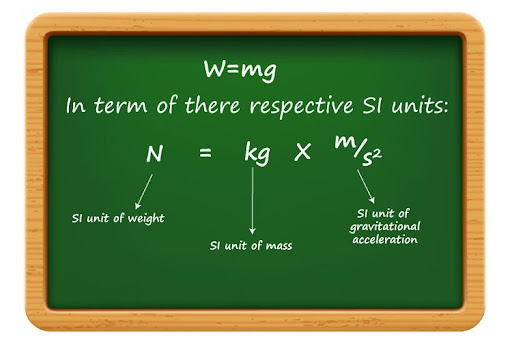# State five differences between mass and weight?

I would like to address this question of yours in a somewhat rigorous manner and draw a more conceptual comparison between the two - and perhaps there’s no better way to start off than from their definitions!

1. They are defined differently

Mass is defined as the amount of matter present within a body; moreover, it is a measure of the amount of inertia that an object possesses (Inertia in itself is a property of any object that makes it resist any change in its state of rest or motion).

On the other hand, weight is defined as the amount of gravitational force that is exerted on an object.

This takes us directly to the next fundamental difference:

2. Mass is a scalar, Weight is a vector
Because mass is just the amount of matter, it is a scalar quantity, measured in SI unit kilograms (kg) - meaning it needs only a magnitude in order to be defined completely.

This is starkly different from weight: because it is a gravitational force (with SI unit of Newton (N)), it is actually a vector - meaning it needs both a magnitude and direction in order to be expressed fully.

Just to put into perspective: it makes perfect sense to say that the apple that fell before Newton did so because of its weight acting downwards - but to say that its mass was also ‘acting downwards’ would be a meaningless claim!

3. Mass is a base quantity, Weight is a derived quantity

We’ve mentioned the SI unit that expresses both mass and weight as quantities, we have yet to make a comparison on the type of quantities that they are!

Mass is a base quantity, meaning it belongs to the family of fundamental quantities (like time and length, for instance) that cannot be expressed in terms of another quantity.

But weight is a derived quantity, meaning it in itself can be written in terms of other base quantities - and that is obvious from the equation we use to calculate weight:

W = mg

In fact, you can even prove that weight can be written in terms of other base quantities!Notice how we’ve broken down the Newton as simply kgm/s2!
I would like to take this moment to highlight an important point: while mass and weight are different kinds of quantities entirely, keep in mind that weight in itself depends on mass. In other words, you cannot have weight if you don’t have mass!
4. Mass is constant, weight can vary
Because mass is a measure of the amount of matter, we quickly put two and two together and realize that mass cannot change unless you remove or add matter within an object. But weight is starkly different, because it can change depending on the amount of gravitational force acting on an object.
To put this into perspective, let’s go through a thought experiment: let’s pick up the same apple that fell in front of Newton all those years ago. Suppose you launch it up to space (perhaps place it in the same orbit that Sputnik 1 followed!). You’ll notice that because the apple is so high up - and hence so far away from the Earth’s gravitational influence - you’ll notice that the apple will feel lighter. That’s because its weight has decreased due to a lesser amount of gravitational force acting on the apple!
But assuming that you resist temptation and don’t take a bite out of the apple, the amount of matter it has will not have changed - meaning its mass will remain the same.
5. Mass has no respective field - but weight does!
I would now like to fine tune the previous discussion a bit more through the same thought experiment: suppose that now you’ve driven your (more aptly Newton’s) orbiting apple over to the Moon’s surface. There, you’ll notice once more that the apple feels lighter, and this time it’s because the Moon itself has a gravitational field that is weaker than that of the Earth (In fact, the Moon’s gravitational field is 1/6th that of the Earth!). You’ll also notice that it’s mass will not have changed yet again.
This shows that weight in itself depends on the strength of the gravitational field it is placed in (numerically, it is represented by ‘g’ in the equation we just went through!). You can look at it the other way round as well: i.e. the gravitational field of planets is what’s causing a gravitational force - and hence weight - to exist in the first place. Mass, on the other hand, doesn’t need such a special field or region, simply because such a thing would have no bearing on the amount of stuff it can hold.
I hope now you have a better understanding of how intrinsically - yet interestingly - mass and weight are different from one another!